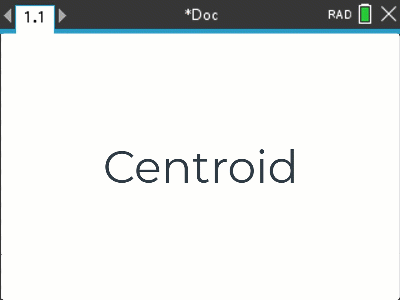# Activities

•• ##### AuthorMiddle
Aust Senior

50 Minutes

• ##### Device
•TI-Nspire™ CX
•TI-Nspire™ CX CAS
• ##### Software

TI-Nspire™
TI-Nspire™ CAS

5.2

## Centroid#### Activity Overview

This activity is aligned with the Circumcentre and Orthocentre activities forming a natural progression for the Euler line activity. Students calculate gradients, equations to lines, solve equations simultaneously and use the calculator's tools to check their answers.

#### Objectives

The aim of this activity is to put a range of coordinate geometry skills into context, including midpoints, equations to lines (given two points) and simultaneous equations. The mixture of skills and processes helps students build and connect these skills.

#### Vocabulary

• Midpoint
• Straight line equation
• Simultaneous equations
• Distance between two points
• Similar triangles
• Geometric proof